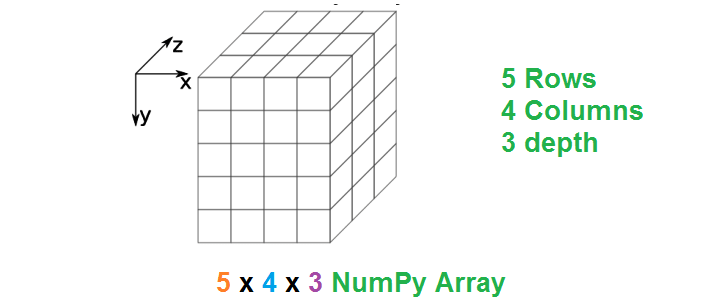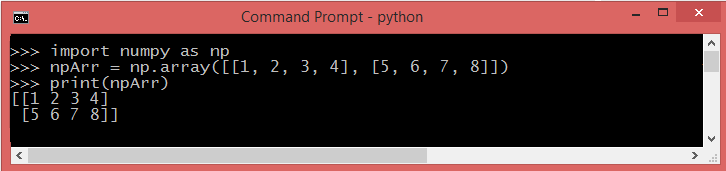# NumPy - Numerical Python

NumPy, short for Numerical Python, serves as a swift and adaptable library designed for the Python programming language. It enriches Python with the ability to handle extensive N-dimensional arrays and matrices, supplemented by an extensive assortment of comprehensive mathematical functions that efficiently operate on these multidimensional arrays. Conceived in 2005 by Travis Oliphant, NumPy has emerged as a cornerstone package for scientific computing in Python. Notably, NumPy arrays are stored in contiguous memory locations, in contrast to Python lists that dynamically grow, enabling rapid and efficient access and manipulation of these arrays during processes.## How To Install NumPy

### Prerequisites

1. Python installed on your system

The most straightforward method to install NumPy is through the use of Pip (Pip Installs Packages). If Pip is not already installed on your system, you will need to configure the package manager that matches the version of Python you are using.

With Pip set up, you can use its command line for installing NumPy .

pip3 install numpy

## Importing the NumPy module

>>> import numpy

When dealing with a substantial number of calls to NumPy functions, repeatedly writing numpy.x() can be cumbersome and make the code less readable. As a common practice, developers frequently import the library under the shorter alias np. This allows them to use the NumPy functions with a more concise syntax, such as np.x(), significantly improving code clarity and reducing typing effort.

>>> import numpy as np

## Create a NumPy array

Creating a 1-D NumPy array with continuous 9 values.

>>> import numpy as np >>> npArr = np.array([1, 2, 3, 4, 5,6,7,8,9]) >>> print(npArr) [1 2 3 4 5 6 7 8 9]

### Check the type of NumPy array

>>> print(type(npArr)) <class 'numpy.ndarray'>

### Create a 2-D NumPy array

Creating a 2-D NumPy array with 2 rows and 4 columns.### Create a 3-D NumPy array

Creating a 3-D NumPy array with 2 rows, 3 columns and 4 depth.

>>> npArr = np.array([[[1, 2, 3,4], [5, 6, 7,8],[9, 10, 11,12]], [[1, 2, 3,4], [5, 6, 7,8],[9, 10, 11,12]]]) >>> print(npArr) [[[ 1 2 3 4] [ 5 6 7 8] [ 9 10 11 12]] [[ 1 2 3 4] [ 5 6 7 8] [ 9 10 11 12]]]

## NumPy Array Shape

The shape property of a NumPy array returns a tuple with the size of each array dimension.

### 1-D NumPy Array shape

>>> import numpy as np >>> npArr = np.array([1, 2, 3, 4, 5,6,7,8,9]) >>> npArr.shape (9,)

Here npArr is a 1-D NumPy array so the shape of the array is (9,), this means that the array has continuous 9 values only.

### 2-D NumPy Array shape

>>> npArr = np.array([[1, 2, 3, 4], [5, 6, 7, 8]]) >>> npArr.shape (2, 4)

Here npArr is a 2-D NumPy array so the shape of the array is (2, 4), this means that the array has 2 rows and 4 columns dimensions.

### 3-D NumPy Array shape

>>> import numpy as np >>> npArr = np.array([[[1, 2, 3, 4], [5, 6, 7,8],[9, 10, 11,12]], [[1, 2, 3,4], [5, 6, 7,8],[9, 10, 11,12]]]) >>> npArr.shape (2, 3, 4)

Here npArr is a 3-D NumPy array so the shape of the array is (2, 3, 4), this means that the array has 2 rows, 3 columns and 4 depth dimensions.

### Conclusion

NumPy, short for Numerical Python, is a fast and versatile library for the Python programming language. It enables support for large N-dimensional arrays and matrices, along with a comprehensive collection of mathematical functions for efficient data manipulation in scientific computing.